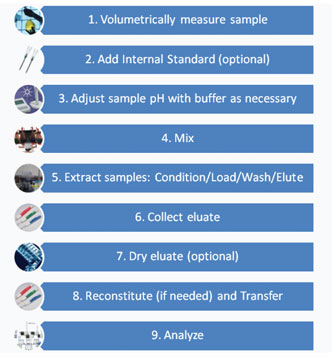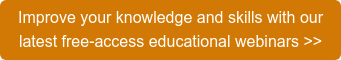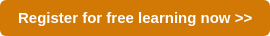# Liquid Chromatography

We’ve covered many of the types of sample preparation options available to make samples ready for instrumental analysis by gas or liquid chromatography and mass spectrometry detection. There are techniques such as QuEChERS, liquid-liquid extraction (LLE) and solid-supported liquid extraction (SLE) that offer a straightforward means of getting a sample into a state that is ready to be analysed.There are also sample prep techniques that provide an extra level of cleanup that enable you to see low concentrations of your analytes while also removing background contaminants that are not of interest or that may interfere with your analysis. These sample prep options — including solid-phase extraction (SPE), solid-phase microextraction (SPME) and online SPE, plus others — are ideal for when you need extra selectivity from your sample prep.

Even if you’ve selected the sample prep process that is just right for your needs and you have an instrument method optimized to deliver the performance you need from your application, an entire day’s effort can be lost if there was an error made in the pre-preparation process. Common sources of error that occur in the pre-preparation include sample mix-ups, incorrectly prepared calibration curves or failure to add internal standards where needed. Additionally, the reported concentrations of any quantitative work depend on the accuracy of the sample quantity used.

Here we will look at measurement techniques that contribute directly to the precision and accuracy of your application. There are many options available in terms of pipettes, analytical balances, graduated cylinders and other tools for measuring, filling, aliquotting, and so forth. Selecting from among these options is not always clear. Selecting the right tool with the right level of accuracy for the specific step in the pre-prep process can help save time and money, so we’ll explore how to select the right measurement tool for the right part of the sample prep process, with some tips and tricks for increasing efficiency and accuracy in your sample prep process.

Where (and When) Do You Need to Be Accurate in Sample Prep?
In general, a higher level of accuracy in pre-sample prep is important when you are doing quantitative analysis, that is, identifying and quantifying compounds. One of the first rules of quantitative analysis is that if the volume or mass measurement will directly affect the result, the measurement should be made to the level of accuracy required by your reporting needs, (i.e. the number of significant digits to be reported). To accurately quantify compounds in the sample, the sample needs to be accurately measured. Qualitative methods typically require less accuracy throughout the process but quantitative analysis depends heavily on accuracy and precision at the right points in the method. Figure 1 represents a basic sample preparation process using a solid-phase extraction (SPE) cleanup method. We’ll use this cleanup approach to illustrate opportunities in the sample prep process where more (or less) accuracy is required.Figure 1: Representative solid-phase extraction (SPE) sample preparation process.

In a solid-phase extraction (SPE) sample preparation method, there are three areas where attention to accuracy is critical — measuring out the liquid sample (Step 1), creating the calibration standards and any quality control samples, and adding the internal standard (Step 2). If the method you are using does not call for an internal standard, you will also need to have accuracy in Step 8, reconstituting the extract, to be able to calculate concentrations of any compounds that are found.

Why Does Pre-Prep Accuracy Matter?
To illustrate the effects on a calculated concentration, we can use two different formulas based on the calibration curve types. If we wanted to quantify a target compound in a volume of liquid using an external standardization calibration method and a chromatographic method where response gives an area count, we can use the following equation to calculate the concentration (1):

Where
C = concentration of the analyte
AS = Area (or height) count of the sample compound peak
Vt = Total volume of the final extract
D = Dilution factor, if applicable
Vi = Injection volume
VS = Volume of the sample used
CF = Mean calibration response factor, calculated from the calibration curve

Using this equation, a 5% error in the sample volume leads to a 5% change in the calculated amount. The more inaccurate the sample volume measurement, the more inaccurate the calculated amount would be. This is particularly true if you cannot correct for the measurement error, because you don’t know one was made.

Using an internal standard helps take out some of this room for error (one of the many advantages of using an internal standard). With an internal standard, the final sample extract volume is not a factor in the concentration calculations (adapted from 1):

Where
C = Concentration of the analyte
AS = Area or height count of the sample compound peak
CIS = Concentration of internal standard
VI = Injection volume
D = Dilution factor, if applicable
AIS = Area or height count of the internal standard peak
RF = Average calibration response factor, calculated from the calibration curve
VS = Total volume of the sample used.

So, knowing that the sample measurement is an important aspect of pre‑preparation, what can be done to improve accuracy?### Posts by Topic